Courses

# Test: Electrochemistry 1 - From Past 28 Years Questions

## 23 Questions MCQ Test Chemistry 28 Years Past year papers for NEET/AIPMT Class 12 | Test: Electrochemistry 1 - From Past 28 Years Questions

Description
This mock test of Test: Electrochemistry 1 - From Past 28 Years Questions for Class 12 helps you for every Class 12 entrance exam. This contains 23 Multiple Choice Questions for Class 12 Test: Electrochemistry 1 - From Past 28 Years Questions (mcq) to study with solutions a complete question bank. The solved questions answers in this Test: Electrochemistry 1 - From Past 28 Years Questions quiz give you a good mix of easy questions and tough questions. Class 12 students definitely take this Test: Electrochemistry 1 - From Past 28 Years Questions exercise for a better result in the exam. You can find other Test: Electrochemistry 1 - From Past 28 Years Questions extra questions, long questions & short questions for Class 12 on EduRev as well by searching above.
QUESTION: 1

### The equilibrium constant of the reaction: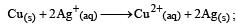E° = 0.46 V at 298 K is 

Solution: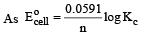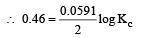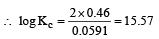or  Kc = Antilog 15.57 = 3.7 × 1015 ≈ 4 × 1015

QUESTION: 2

### On the basis of the following E° values, the strongest oxidizing agent is :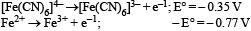Solution:

For the strongest oxidizing agent, the oxidizing potential should be least. Here, the oxidizing potential of Fe+2 is less than that of [Fe(CN)6​]4−. Therefore, Fe+2 is stronger oxidizing agent than [Fe(CN)6​]4−. Also, the stronger oxidizing agent should easily reduce itself. Here, Fe+3 is easily reduced than Fe+2. Therefore, among all the four, Fe+3 is the stronger oxidizing agent.

QUESTION: 3

### Kohlrausch’s law states that at : 

Solution:

Kohlrausch ’s Law states that at infinite dilution, each ion migrates independently of its co-ion and contributes to the total equivalent conductance of an eletrolyte a definite share which depends only on its own nature.
From this definition we can see that option (d) is the correct answer.

QUESTION: 4

Standard free energies of formation (in kJ/mol) at 298 K are – 237.2, – 394.4 and – 8.2 for H2O(l), CO2(g) and pentane (g), respectively. The value E°cell for the pentane-oxygen fuel cell is :  

Solution:

Writing the equation for pentane-oxygen fuel cell at respective electrodes and over all reaction, we get At Anode: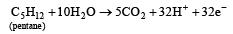At Cathode: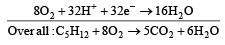Calculation of ΔG° for the above reaction

ΔG° = [5×(–394.4) + 6× (–237.2)]
– [–8.2]
= – 1972.0 – 1423.2 + 8.2 = – 3387.0 kJ
= – 3387000 Joules.
From the equation we find n = 32

Using the relation,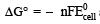and substituting various values, we get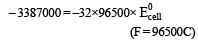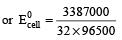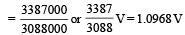Thus option (c) is correct answer.

QUESTION: 5

Given: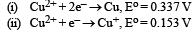Electrode potential, Eo for the reaction,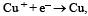will be :

Solution: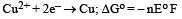= – 2 × F × 0.337
= – 0.674 F        ....(i)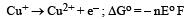= – 1 × F × – 0.153
=  0.153 F     ....(ii)

On adding eqn (i) & (ii)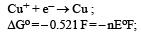Here n = 1       Δ Eo = + 0.52 V

QUESTION: 6

Al 2O3 is reduced by electrolysisat low potentials and high currents. If  4.0 × 104 amperes of current is passed through molten Al2O3 for 6 hours, what mass of aluminium is produced? (Assume 100% current efficiency. At. mass of Al = 27 g mol–1) 

Solution: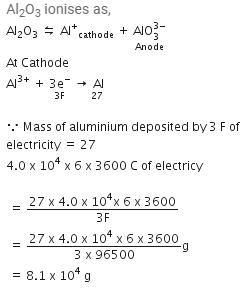Now since 96500 C liberates 9 g of Al

8.64 × 108  C liberates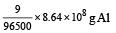= 8.1 × 104 g of Al

QUESTION: 7

The equivalent conductance of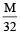solution ofa weak monobasic acid is 8.0 mhos cm2 and at infinite dilution is 400 mhos cm2. The dissociation constant of this acid is: 

Solution:

Degree of dissociation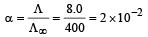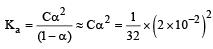= 1.25 x 10-5

QUESTION: 8

For the reduction of silver ions with copper metal, the standard cell potential was found to be + 0.46 V at 25°C. The value of standard Gibbs energy, ΔG0 will be (F = 96500 C mol –1)

Solution: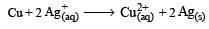Here, n = 2 ,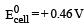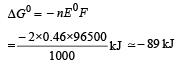QUESTION: 9

An increase in equivalent conductance of a strong electrolyte with dilution is mainly due to: 

Solution:

Dilution of strong electrolytes increases ionisation, hence ionic mobility of ions which in turn increases equivalent conductance of the solution.

QUESTION: 10

Which of the following expressions correctly represents the equivalent conductance at infinite dilution of Al2 (SO4)3, Given that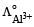and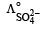are the equivalent conductances at infinite dilution of the respective ions? 

Solution:

Equivalent conductance of an electrolyte at infinite dilution is given by the sum of equivalent conductances of the respective ions at infinite dilution.
∴ Correct choice : (c)

QUESTION: 11

Consider the following relations for emf of a electrochemical cell:                           
(i) emf of cell = (Oxidation potential of anode) – (Reduction potential of cathode)
(ii) emf of cell = (Oxidation potential of anode) + (Reduction potential of cathode)
(iii) emf of cell = (Reduction potential of anode) + (Reduction potential of cathode)
(iv) emf of cell = (Oxidation potential of anode) – (Oxidation potential of cathode)
Which of the above relations are correct?

Solution:

Option (b) and (d) are correct
∴ Correct choice : (a)

QUESTION: 12

Standard electrode potential of three metals X, Y and Z are – 1.2 V, + 0.5 V and – 3.0 V, respectively. The reducing power of these metals will be : 

Solution:

As the value of standard reduction potential decreases the reducing power increases i.e.,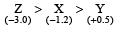QUESTION: 13

The electrode potentials for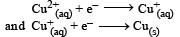are + 0.15 V and + 0.50, respectively. The value of E° Cu 2+ / Cu will be :

Solution: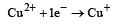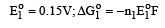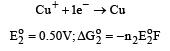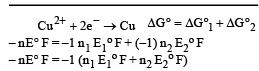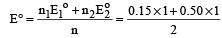⇒ 0.325

QUESTION: 14

Standard electrode potential for Sn4+ / Sn2+ couple is + 0.15 V and that for the Cr3+ / Cr couple is – 0.74 V. These two couples in their standard state are connected to make a cell. The cell potential will be : 

Solution: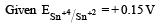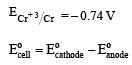= 0.15 – (– 0.74)
= + 0.89 V

QUESTION: 15

If the E°cell for a given reaction has a negative value, then which of the following gives the correct relationships for the values of ΔG° and Keq ?

Solution:

Standard Gibbs free energy is given as ΔG°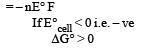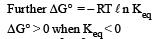QUESTION: 16

A solution contains Fe2+, Fe3+ and Iions. This solution was treated with iodine at 35°C. E° for Fe3+ / Fe2+ is + 0.77 V and E° for I2/2I = 0.536 V.The favourable redox reaction is : [2011 M]

Solution: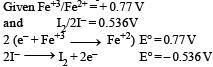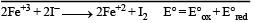= 0.77 – 0.536
= 0.164 V
So, reaction will taken place.

QUESTION: 17

Limiting molar conductivity of NH4OH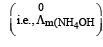is equal to : 

Solution: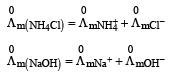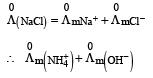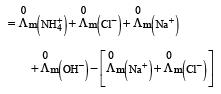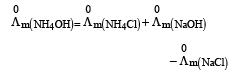QUESTION: 18

Molar conductivities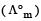at infinite dilution of NaCl, HCl and CH3COONa are 126.4, 425.9 and 91.0 S cm2 mol–1 respectively.for CH3COOH will be : [2012 M]

Solution: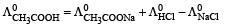= 91 + 425.9 – 126.4 = 390.5

QUESTION: 19

A hydrogen gas electrode is made by dipping platinum wire in a solution of HCl of pH = 10 and by passing hydrogen gas around the platinum wire at one atm pressure. The oxidation potential of electrode would be ? [NEET 2013]

Solution: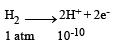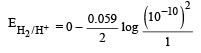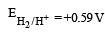QUESTION: 20

At 25°C molar conductance of 0.1 molar aqueous solution of ammonium hydroxide is 9.54 ohm-1 cm2mol-1 and at infinite dilution its molar conductance is 238 ohm-1 cm2 mol-1. The degree or ionisation of ammonium hydroxide at the same concentration and temperature is :[NEET 2013]

Solution: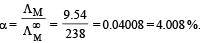QUESTION: 21

A button cell used in watches function s as following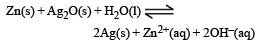If half cell potentials are :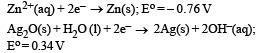The cell potential will be : [NEET 2013]

Solution:

Cell = E°OP + E°RP = 0.76 + 0.314 = 1.10 V

QUESTION: 22

How many grams of cobalt metal will be deposited when a solution of cobalt (II) chloride is electrolyzed with a current of 10 amperes for 109 minutes (1 Faraday = 96,500 C; Atomic mass of Co = 59 u) [NEET Kar. 2013]

Solution:

Applying,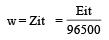Equivalent weight of cobalt (II) = 59/2
I = 10 A
Time (t) = 109 min = 109 × 60 sec
Substituting these values we get,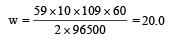QUESTION: 23

Consider the half-cell reduction reaction :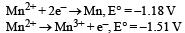The E° for the reaction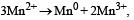and possibility of the forward reaction are, respectively [NEET Kar. 2013]

Solution:

ΔE° =  E°red + E°oxd = – 1.81 – 1.51
= – 2.69
Since ΔE° is negative
∴    ΔG = –nFE°, ΔG will have positive value so, forward reaction is not possible.